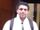## How to calculate average

Hi ,

I have a dataset which has item id , item type , some factors and i want to calculate average by item id and item type

 Item id Item_type APPLE_T1 ORANGE_T1 123 BOTH 2 2 123 BOTH 2 2 123 BOTH 2 2 123 APPLE 1.6 0 123 ORANGE 0 1.3 456 BOTH 2 2 456 BOTH 2 2 456 APPLE 1.6 0

So while calculating average of APPLE_T1 and Orange_t1 we should consider by itemid and itemtype we should note that Item_type=BOTH means (its applicable for both APPLES AND ORANGES )

So after we create average of those by ids the table should look like below

So for item id 123 - APPLE_T1 1.9 is  (2+2+2+1.6)/4  and for orange_t1 1.825 is  (2+2+2+1.3)/4

and for item 456 - APPLE_T1 is (2+2+1.6)/3  and for orange_t1 = 2+2 /2

 Item id APPLE_T1 ORANGE_T1 123 1.9 1.825 456 1.86 2

Thank you

1 ACCEPTED SOLUTION

Accepted Solutionsnovinosrin
Tourmaline | Level 20

## Re: How to calculate average

data have;
infile datalines truncover;
input Item Item_type \$ APPLE_T1 ORANGE_T1;
datalines;
123 BOTH 2 2
123 BOTH 2 2
123 BOTH 2 2
123 APPLE 1.6 0
123 ORANGE 0 1.3
456 BOTH 2 2
456 BOTH 2 2
456 APPLE 1.6 0
;

proc sql;
create table want as
select Item, mean(case when APPLE_T1=0 then . else APPLE_T1 end ) as m1, mean(case when ORANGE_T1=0 then . else ORANGE_T1 end ) as m2
from have
group by item;
quit;

Also try proc means, summary etc. Search and copypaste code from internet

4 REPLIES 4novinosrin
Tourmaline | Level 20

## Re: How to calculate average

data have;
infile datalines truncover;
input Item Item_type \$ APPLE_T1 ORANGE_T1;
datalines;
123 BOTH 2 2
123 BOTH 2 2
123 BOTH 2 2
123 APPLE 1.6 0
123 ORANGE 0 1.3
456 BOTH 2 2
456 BOTH 2 2
456 APPLE 1.6 0
;

proc sql;
create table want as
select Item, mean(case when APPLE_T1=0 then . else APPLE_T1 end ) as m1, mean(case when ORANGE_T1=0 then . else ORANGE_T1 end ) as m2
from have
group by item;
quit;

Also try proc means, summary etc. Search and copypaste code from internet

It Worked

Thank you

## Re: How to calculate average

So basically you are ignoring the ITEM_TYPE variable and assuming that a value of zero is a missing value instead?

What if zero is a valid value?

``````create table want as
select Item
, mean(case when item_type ne 'ORANGE' then APPLE_T1 else . end) as mean_apple
, mean(case when item_type ne 'APPLE' then ORANGE_T1 else . end) as mean_orange
from have
group by item
;
quit;``````

## Re: How to calculate average

A multilabel format may also be an option, here's how it may work. You'd have to check if its any easier, but I can't seem to think this one through for some reason.

``````data have;
infile datalines truncover;
input Item Item_type \$ APPLE_T1 ORANGE_T1;
datalines;
123 BOTH 2 2
123 BOTH 2 2
123 BOTH 2 2
123 APPLE 1.6 0
123 ORANGE 0 1.3
456 BOTH 2 2
456 BOTH 2 2
456 APPLE 1.6 0
;
run;

title 'Fruit Summary by Item Type';
proc means data=HAVE n mean sum maxdec=2;
class item item_type /mlf;
format item_type \$fruit.;
var apple_t1 orange_t1;
ods output summary=want;
run;``````

Discussion stats
• 4 replies
• 25253 views
• 1 like
• 4 in conversation